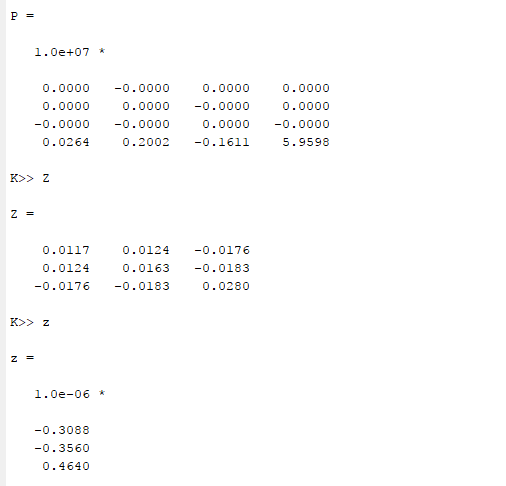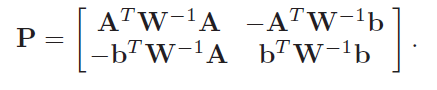# Relaxed SDP problem is infeasible and unbounded

Hi,
I am solving the problem in (16), which is relaxed in (17)
I am getting the following message : “sqlp stop: dual problem is suspected of being infeasible Status: Unbounded
Optimal value (cvx_optval): -Inf,” while I get small values of z, can anyone help me? Attached is the screenshot that explains my question.

Based on the solver status, CVX has concluded that your (relaxed) problem is unbounded. Do you have reason to believe that it is actually not unbounded? You have not provided a reproducible problem. Just from looking at it, depending on the values of your input data, which you have not provided us, it looks like it could be unbounded.

Perhaps someone more knowledgeable than me can assess the reliability of the unboundedness conclusion (dual infeasibility) based on the output.

Thank you Mark for your quick feedback,
I don’t really have reasons of believing that it should not be unbounded, the inputs are basically coming from the matrix P, on the image below is the P matrix, and the corresponding values of Z, z obtained for that simulation.
What properties should P have in order not to have an unbounded problem? Anyone mature in this is welcome to give us feedback.Thanks.

Per (16) and (17), dim = 3, so Z is 4 by 4, which means P needs to be 5 by 5, but you show P as 4 by 4. Does this P actually correspond to dim = 2?

I can’t determine what your P matrix is based on the digits shown - I don’t know what those 0.0000 * 1e+07 and -0.0000e+07 entries are . Are they all really (or should be) exactly zero? Having the largest element be 5.9598e+07 may not be great from a numerical standpoint. If those +/- 0.0000e+07 really should be exactly zero, you could set them to exactly zero and re-scale P by dividing by 1e±7, which will scale the optimal objective value, and perhaps will improve the numerics of the problem.

It would be better if you copy and paste your program and output, and use the Preformatted text icon, rather than using an image. Also, when showing the values of variables, use format long.

Hi again, sorry I forgot to mention, the equation (16) and (17) are for dim=3, but in the simulation I performed, dim=2.
The entries of P should not be zero, they are obtained aswhere W is the error covariance matrix, and matrix A and vector b do not have zero elements in general. Below are the entries of P in the long format, together with those of Z and z.

P =

1.0e+07 *

0.000000093395104 -0.000000536605236 0.000000691468788 0.000017726973530
0.000000086234944 0.000001034750411 -0.000000990203862 0.000012710253773
-0.000000225316090 -0.000000829824337 0.000000596176409 -0.000037361330294
0.026434128918895 0.200200852357165 -0.161069815820987 5.959781703047424

K>> Z

Z =

0.011702435217480 0.012407545631967 -0.017562291904678
0.012407545631967 0.016329276578637 -0.018283756868600
-0.017562291904678 -0.018283756868600 0.028031711796116

K>> z

z =

1.0e-06 *

-0.308811521280786
-0.356047723370061
0.463974074743146

Hi,
I have tried to make the changes in my program. The errors were in the computation of P. Now, the problem is being solved but the last entry of the vector z (dim+1) which is the norm of the z(1:dim) is sometimes positive, other times negative. It should always be positive. Should this be enforced naturally in the program or do I need to insert in a new constraint? A more knownledgeable person is welcome to give me an idea.

Where in your program is there anything which by construction or constraint would make the last entry of the vector z (dim+1) be the norm of the z(1:dim) ?

Of course you are free to add a constraint to constrain this entry to be non-negative. Do you have some theoretical basis as to why the last entry of the vector z (dim+1) should be the norm of the z(1:dim)? If it should theoretically, but then you have to force it to be non-negative, that’s a sign that something is wrong, so “covering it up” by brute force addition of a constraint might not be a good idea.

Thank you very much Mark for your support! Above I show also the constrained weighted LS problem (with a non convex constrain) in equation 15. The SP program is formulated in eq. 16 and the relaxed in eq. 17. The constraint in eq.15 indicates that last entry of z must be positive, and this constraint is changed into the first constraint of eqs. 16 and 17. Do we lose the meaning of the constraint in eq. 15 by formulating the SDP? If yes, then the question is to see how to keep that constrain meaning.

You solved a relaxation, not the original problem. If it is not the case that Z = z*z’ for the optimal solution to the relaxation, then you have lost something in the process. The best way to deal with that is really a modeling issue outside the scope of this forum.

The equality Z=z*z’ in the optimal solution is fulfilled. Thanks for your inputs .